Courses

# Summary - Moment of Inertia Mechanical Engineering Notes | EduRev

## Mechanical Engineering : Summary - Moment of Inertia Mechanical Engineering Notes | EduRev

The document Summary - Moment of Inertia Mechanical Engineering Notes | EduRev is a part of the Mechanical Engineering Course Engineering Mechanics - Notes, Videos, MCQs & PPTs.
All you need of Mechanical Engineering at this link: Mechanical Engineering

Moment of Inertia

The Second moment of area, also known as moment of inertia of plane area is a geometrical property of an area which reflects how its points are distributed with regard to an arbitrary axis.

Moment of Inertia is given as the sum of the products of each area in the lamina with the square of its distance from the axis of rotation.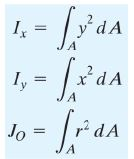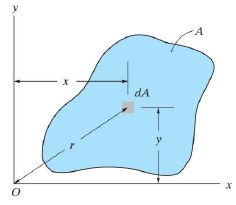It is denoted with I for an axis that lies in the plane or with a J for an axis perpendicular to the plane (referred as polar moment of inertia).

 Characteristics of Moment of Inertia MI is determined with reference to an axis and changes with change in axis position or orientationMoment of Inertia about any axis is always a positive quantityMI is minimum when the axis of rotation passes through the centroidThe SI units for area moments of inertia are expressed as m4 or mm4.

Parallel Axis Theorem

Parallel Axis Theorem states that the moment of inertia of a plane lamina about any axis is equal to the sum of moment of inertia about a parallel axis passing through the centroid and the product of area and square of the distance between the two parallel axes.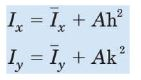Proof

In Figure, the x0-y0 axes pass through the centroid C of the area. If I’x and I’y are the moments of inertia of the area about the centroidal axes x0-y0 and Ix and Iy the moments of inertia of the area about the parallel axes x-y.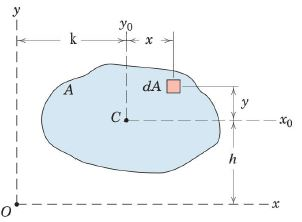By definition, the moment of inertia of the element dA about the x-axis is given by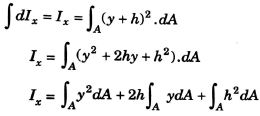Since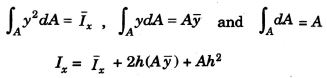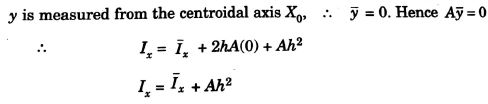Similarly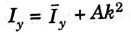Perpendicular Axis Theorem

Perpendicular Axis Theorem states that the moment of inertia of a plane lamina about an axis perpendicular to the lamina and passing through the centroid is equal to the sum of moment of inertias of the lamina about two mutually perpendicular axes in the same plane and passing through the centroid.

OR

Perpendicular Axis Theorem states that the polar moment of inertia is equal to the sum of moment of inertias of the two centroidal axis in the plane of the lamina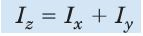Proof

In Figure, the x0-y0 axes pass through the centroid C of the area. If I’x and I’y are the moments of inertia of the area about the centroidal axes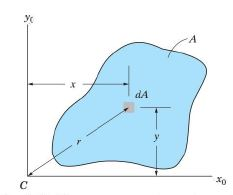We know that by defination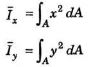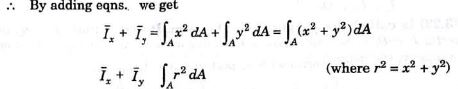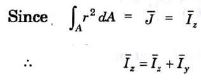Moment Of Inertia Of Regular Sections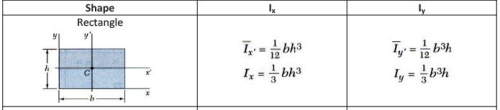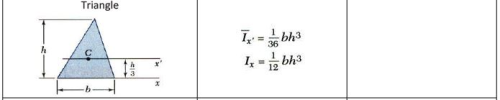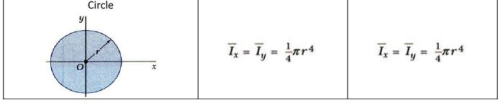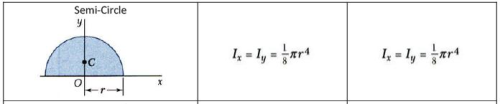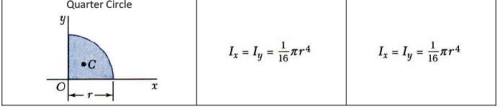A. MOMENT OF INERTIA OF COMPOSITE SECTIONS

 Procedure 1. Divide the given area into its simpler shaped parts.2. Locate the centroid of each part and indicate the perpendicular distance from each centroid to the desired reference axis.3. Determine the MI of each “simpler” shaped part about the desired reference axis using the parallel-axis theorem4. The MI of the entire area about the reference axis is determined by performing an algebraic summation of the individual MIs obtained

Polar Moment of Inertia

The moment of inertia of a plane lamina about an axis perpendicular to the lamina and passing through the centroid is called polar moment of inertia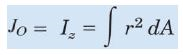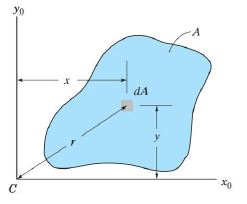The radius of gyration of an area about an axis is the distance to a long narrow strip whose area is equal to the area of the lamina and whose moment of inertia remain the same as that of the original area

Consider a lamina of area A whose moment of Inertia is Ix, Iy and Iz with respect to x, y and z axis.
Imagine the area is concentrated into a thin strip parallel to the axis and has the same moment of inertia as that of the lamina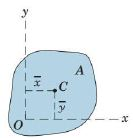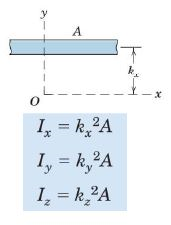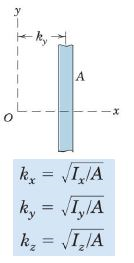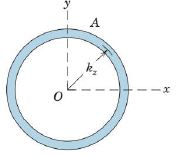Product of Inertia

The product of inertia is the sum of the product of each differential area and its moment arms about the two perpendicular reference axis.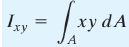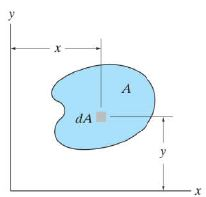Characteristics of Product of Inertia  The SI units for product of inertia are expressed as m4 or mm4.Product of inertia may be positive, negative, or zero, depending on the location and orientation of the coordinate axes.Product of inertia for an area will be zero if either x or y axis is an axis of symmetry for the area.

Rotation of Moments of Inertia

The moment of inertia and product of inertia of a lamina with respect to an inclined axis u and v rotated an angle θ with respect to the reference axis x- y is given by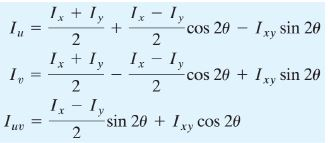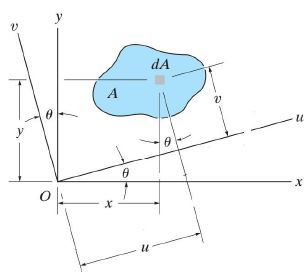Principal Axes and Principal moments of Inertia

The inclined set of axis u-v about which the product of inertia Ixy is zero is called the Principal Axes.
The angle made by the principal axes with respect to the reference axes is called principal angle θp.
The moments of inertia about the principal axes are called Principal Moment of Inertia and yield the maximum and minimum values of moment of inertia of the lamina.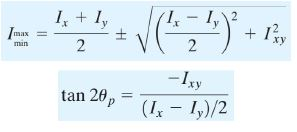Mass moment of Inertia Mass

moment of inertia or rotational inertia of a body is a measure of the body’s resistance to angular acceleration. Mass Moment of Inertia is the sum of the products of the mass of each particle in the body with the square of its distance from the axis of rotation.
The mass moment of inertia about the axis O-O is given as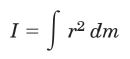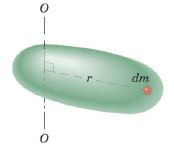Mass Moment of Inertia of Circular Thin Disc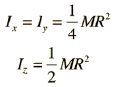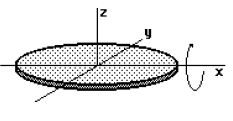Mass Moment of Inertia of Cylinder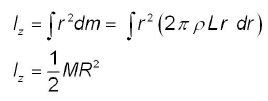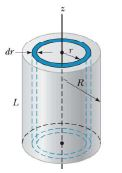Offer running on EduRev: Apply code STAYHOME200 to get INR 200 off on our premium plan EduRev Infinity!

,

,

,

,

,

,

,

,

,

,

,

,

,

,

,

,

,

,

,

,

,

;